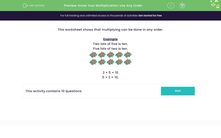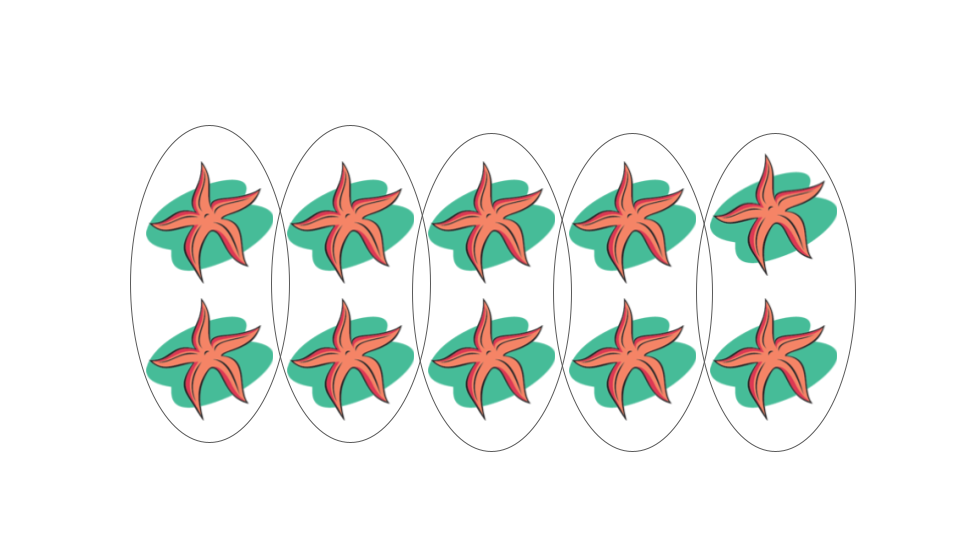# Know Your Multiplication: Use Any Order

In this worksheet, students notice how, for example, 2 x 4 has the same answer as 4 x 2.Key stage:  KS 1

Curriculum topic:   Number: Multiplication and Division

Curriculum subtopic:   Understand Order for Multiplication/Division

Difficulty level:#### Worksheet Overview

In this activity, we will be looking at how multiplication can be done in any order.

This array works in two different ways.

If we group the starfish into columns we have five lots of two and there are 10 starfish altogether.

This is 5 x 2 = 10If we group the starfish into rows we have two lots of five and there are still 10 starfish altogether.

This is 5 x 2 = 10Example

What is 3 times 2?The array above shows 3 x 2 =

To work out what the answer is we can count all the starfish to find the total. There are 6 starfish here so 3 times 2 is 6.

### What is EdPlace?

We're your National Curriculum aligned online education content provider helping each child succeed in English, maths and science from year 1 to GCSE. With an EdPlace account you’ll be able to track and measure progress, helping each child achieve their best. We build confidence and attainment by personalising each child’s learning at a level that suits them.

Get started